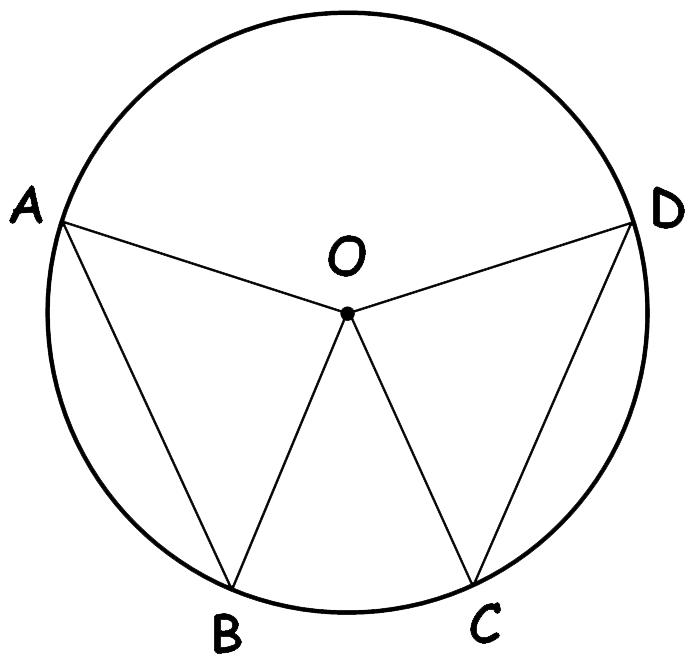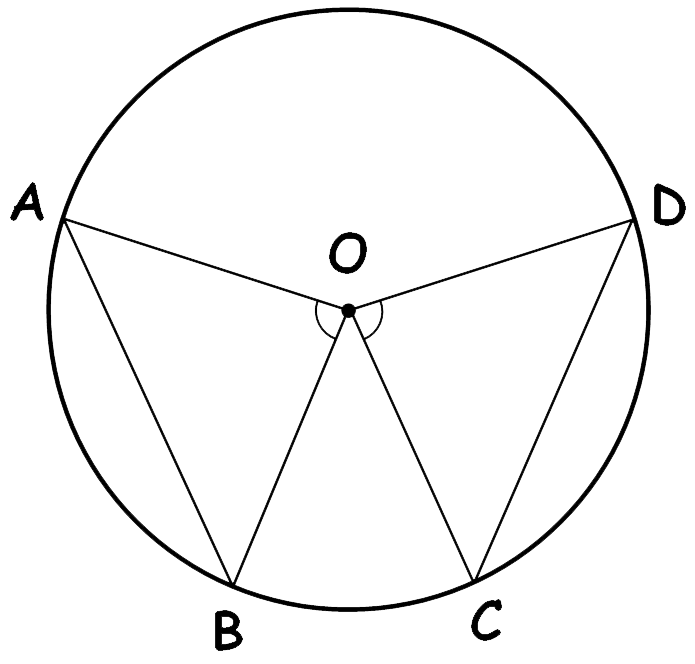# vs.eyeandcontacts.com

## Chapter 10 Circles Exercise 10.2

Question 1: Recall that two circles are congruent if they have the same radii. Prove that equal chords of congruent circles subtend equal angles at their centres.In ∆ AOB and ∆ DOC
OA = OC    (Radii of the circle)
OB = OD    (Radii of the circle)
AB = CD    (Given)

Therefore, ∆ AOB ≅ ∆ COD (SSS rule). By CPCT, ∠AOB = ∠COD

Question 2: Prove that if chords of congruent circles subtend equal angles at their centres, then the chords are equal.